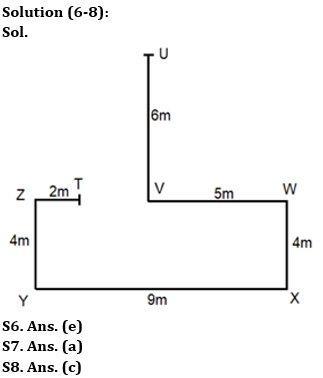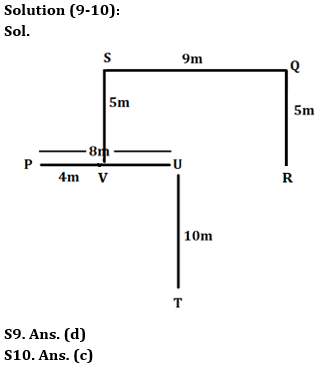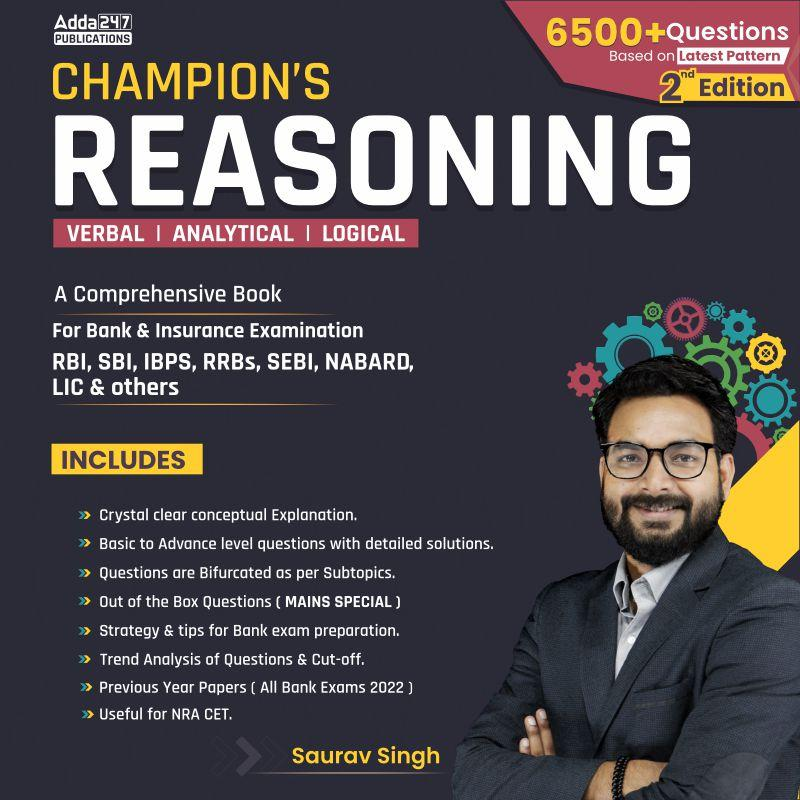Latest Banking jobs   »   IBPS reasoning

# Reasoning Quiz For IBPS PO Prelims 2023 -06th August

Directions (1-5): Study the following arrangement carefully and answer the questions

Y 7 & * M W 2 @ V 1 N % S L ? + 9 8 A \$ C # H J ^ O

Q1. How many alphabets are immediately preceded by an even number?
(a) None
(b) Two
(c) One
(d) Three
(e) More than three

Q2. What is the sum of 3rd number from left end and 2nd number from right end?
(a) 19
(b) 13
(c) 10
(d) 12
(e) 18

Q3. How many vowels are right to the L?
(a) None
(b) Two
(c) One
(d) Three
(e) More than three

Q4. How many symbols are immediately preceded and immediately followed by odd number?
(a) One
(b) Two
(c) Three
(d) Four
(e) None of the above

Q5. Which of the following element is 7th to the left of 13th element from right end?
(a) V
(b) @
(c) W
(d) 2
(e) None of these

Directions (6-8): Answer the questions based on the information given below.
Nitin started walking from point U in south direction. After walking 6m he turns left from point V and walks for 5m till point W. He then turns right and walks for 4m till point X and then turns right and walks for 9m till point Y. He then turns right and walks for 4m till point Z and then turns right and walks for 2m till point T.

Q6. What is the direction of T with respect to W?
(a) North-east
(b) North-west
(c) South-east
(d) South-west
(e) West

Q7. What is the direction of U with respect to Y?
(a) North-east
(b) North-west
(c) South
(d) South-west
(e) West

Q8. What is the shortest distance between point T and W?
(a) 6m
(b) 9m
(c) 7m
(d) 10m
(e) None of these

Directions (9-10): Answer the questions based on the information given below.
Seven points P, Q, R, S, T, U and V are drawn on sheet of paper. S is 5m north of V. T is 10m south of U. Q is 5m north of R. P is 8m west of U. V is 4m east of P. Q is 9m east of S.

Q9. What is the shortest distance between P and R?
(a) 10m
(b) 11m
(c) 12m
(d) 13m
(e) None of these

Q10. What is the direction of T with respect to S?
(a) North-east
(b) North-west
(c) South-east
(d) South-west
(e) None of these

Solutions

Solution (1-5):
S1. Ans. (c)
Sol. Given series: Y 7 & * M W 2 @ V 1 N % S L ? + 9 8 A \$ C # H J ^ O
Hence, there is one alphabet which is immediately preceded by an even number

S2. Ans. (c)
Sol. Given series: Y 7 & * M W 2 @ V 1 N % S L ? + 9 8 A \$ C # H J ^ O
3rd number from left end = 1
2nd number from right end = 9
So, require sum = 9 + 1 = 10

S3. Ans. (b)
Sol. Given series: Y 7 & * M W 2 @ V 1 N % S L ? + 9 8 A \$ C # H J ^ O
Hence, there are two vowels (A, O) to the right of L.

S4. Ans. (e)
Sol. Given series: Y 7 & * M W 2 @ V 1 N % S L ? + 9 8 A \$ C # H J ^ O
Hence, there is no symbol which is immediately preceded and immediately followed by odd number.

S5. Ans. (d)
Sol. Given series: Y 7 & * M W 2 @ V 1 N % S L ? + 9 8 A \$ C # H J ^ O
13th element from the right end = L
So, 7th to the left of L = 2.## FAQs

### When will the IBPS PO prelims 2023 be conducted?

IBPS PO Prelims will be conducted on 23, 30 September, and 1 October 2023.

#### Congratulations!Union Budget 2023-24: Free PDF